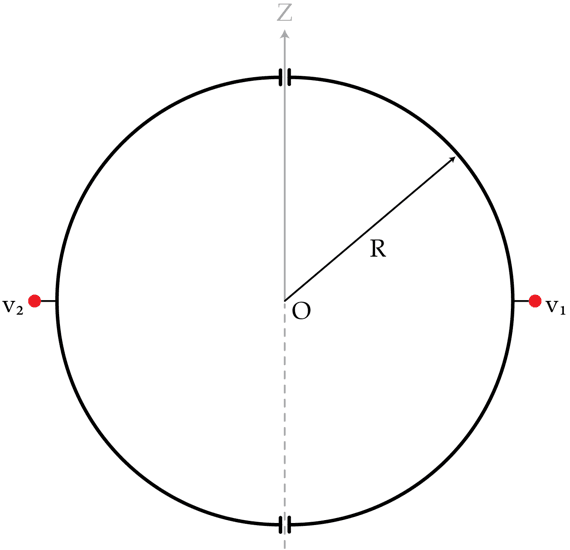# Fixed potentials

Two hemispheres of radius $R=30~\text{cm}$ are held at constant potentials $V_{1}=3~\text{V}$ and $V_{2}=9~\text{V}$. Determine the electric potential in volts at a point P with coordinates $(x,y,z)=(0,0, 3R)$.×